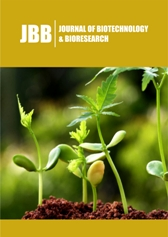• Submissions

### Application of NIR Spectroscopy to Evaluate the Soil Fertility at the Field Scale

Submission: November 06, 2020; Published: December 09, 2020#### Abstract

The Near Infrared Reflectance Spectroscopy (VIS-NIRS) allows for quick analysis of soil abundance in basic macronutrients and development of fertilizer application maps. This article compares the results for pH and soil abundance in available forms of phosphorus, potassium and magnesium estimated using near-infrared spectroscopy in the 580-1850nm range and on the basis of standard laboratory methods. Soil samples were taken from a field of 20.6ha, from the surface of each polygon defined by a grid of squares with sides of 50x50m. The total soil sample (80) assigned to the square 50x50m taken from the arable layer to a depth of 0.2m. The constructed models must be then subjected to validation using an independent set of samples. In the presented experiment the samples were randomly divided into two equal sets, 40 samples each. A simple correlation analysis was conducted in order to estimate the correlations between the results obtained using standard methods and the spectrophotometry. The results were shown high relationship between values obtained by using NIRS and laboratory methods. Coefficients of determination computed for model calibration on the basis of linear regression, which for the soil pH, K content and subsequently for Mg and P was obtained, respectively: R2=0.90; R2=0.91; R2=0.83; R2=0.86. The results show the lower coherence of NIRS results with laboratory results for pH and K2O, followed by Mg and P2O5 for model verification. Determination coefficients in this case were as follows: R2=0.56; R2=0.54; R2=0.51 and R2=0.48. The fertility maps developed on the basis of laboratory analyses and by means of InfraXact™ device differed by 19% for phosphorus and 7,25% (relative root square error for area of fertility classes) for potassium.

Keywords: VIS-NIRS; P; K; Mg; Soil fertility; Abundance maps

﻿

We at Crimson Publishing are a group of people with a combined passion for science and research, who wants to bring to the world a unified platform where all scientific know-how is available read more...